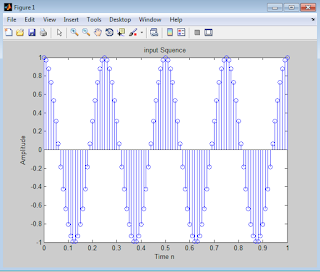Impact-Site-Verification: dbe48ff9-4514-40fe-8cc0-70131430799e

## Search This Blog

Cosine Wave:
Cosine waves and sine waves are identical except for a phase or time shift of 90 degrees, which means 90/360 (or one fourth) of the basic waveform. Sine waves have a value of 0 at time=0, and cosine waves have a value of +1 at time=0.

y(t) = A cos (wt + ɸ  )
where;
A, the amplitude, is the peak deviation of the function from its center position.
ω, the angular frequency, specifies how many oscillations occur in a unit time interval, in
ɸ, the phase, specifies where in its cycle the oscillation begins at t = 0.

Cosine Wave can be obtained by using the following formulae
x=cos(2*pi*fi*n)

MATLAB Code:-
Cosine Wave:
T=input ('Input time of the Sinusoidal signal= ');
fi=input ('Input Frequency of the Sinusoidal signal= ');
n =0:0.01:T;
X=cos(2*pi*fi*n);
stem(n,X);
%plot(n,X)
title('input Squence');
xlabel('Time n');
ylabel('Amplitude');
Output waveform:
Input time of the Sinusoidal signal= 1
Input Frequency of the Sinusoidal signal= 4